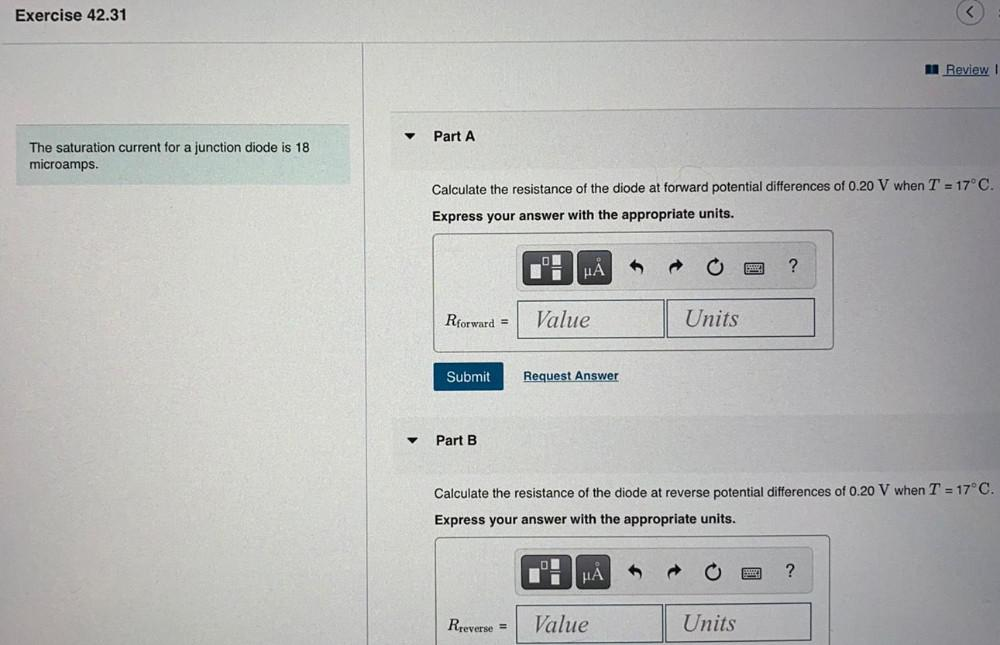Question:

# Exercise 42.31 A Review! Part A The saturation current for a junction diode is 18 microamps. Calculate the resistance of the dioExercise 42.31 A Review! Part A The saturation current for a junction diode is 18 microamps. Calculate the resistance of the diode at forward potential differences of 0.20 V when T = 17°C. Express your answer with the appropriate units. HA ? Rforward = Value Units Submit Request Answer Part B Calculate the resistance of the diode at reverse potential differences of 0.20 V when T = 17°C. Express your answer with the appropriate units. MA ? Rreverse = Value Units